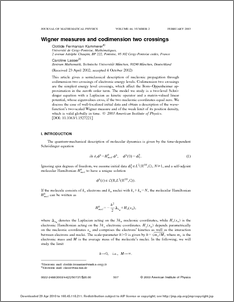Repository: Freie Universität Berlin, Math Department

# Wigner measures and codimension two crossings

Fermanian Kammerer, C. and Lasser, C. (2003) Wigner measures and codimension two crossings. J. Math. Phys., 44 (2). pp. 507-557.Preview

243kB

Official URL: http://dx.doi.org/10.1063/1.1527221

## Abstract

This article gives a semiclassical description of nucleonic propagation through codimension two crossings of electronic energy levels. Codimension two crossings are the simplest energy level crossings, which affect the Born–Oppenheimer approximation in the zeroth order term. The model we study is a two-level Schrödinger equation with a Laplacian as kinetic operator and a matrix-valued linear potential, whose eigenvalues cross, if the two nucleonic coordinates equal zero. We discuss the case of well-localized initial data and obtain a description of the wavefunction’s two-scaled Wigner measure and of the weak limit of its position density, which is valid globally in time.

Item Type: Article Physical Sciences > Physics > Mathematical & Theoretical Physics > Quantum Mechanics Department of Mathematics and Computer Science > Institute of Mathematics 908 Burkhard Schmidt 29 Apr 2010 08:47 03 Mar 2017 14:40

Repository Staff Only: item control page